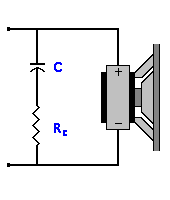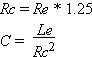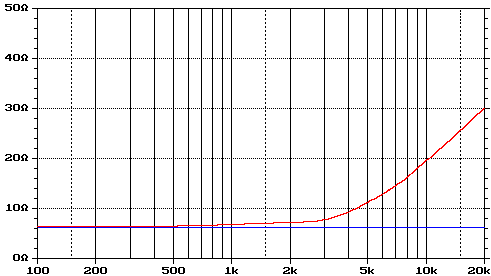# DIY Audio & Video

## Impedance Equalization (Zobel) Circuit Designer / Calculator Help

Impedance Equalization Circuits, also know as Zobel circuits, are used to counteract the rising impedance of a voice coil caused by inductive reactance. This problem mainly occurs in sub woofers because of their large coils. A Impedance Equalization circuit is usually placed after the crossover.

Impedance Equalization Circuit is simply a capacitor (C) and a resistor (Rc) in series, in parallel with the driver.Re = Driver DC Resistance in Ohms

Le = Driver Inductance in mHExample: Say you have a 8 Ohm tweeter with a Re of 5.7 Ohms with a Le of .08 mH The impedance curve for the driver would look like this.

Actual Driver Resistance

Desired Driver ResistanceThe impedance of the tweeter rises because of the voice coils inductance at high frequencies. Using the above formulas, C = 1.58 uF and Rc = 7.13 Ohms. Using the capacitor and resistor will help to flatten out the impedance curve. Experimentation is recommended to achieve the desired result.